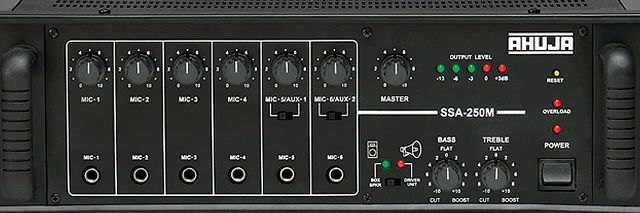## AmplifierThe applications of Op-Amp integrators

From Luke James

Related Vendors

An operational amplifier (op-amp) integrator is an operational amplifier circuit that performs the mathematical operation of integration with respect to time—this means the output voltage is proportional to the input voltage integrated over time.An operational amplifier (often op-amp or opamp) is a DC-coupled high-gain electronic voltage amplifier with a differential input and, usually, a single-ended output. (Source: Unsplash)

To perform mathematical integration, the operational amplifier (op-amp) integrator requires nothing more than an op-amp and a few passive components. Integration can be achieved by using a typical inverting op-amp configuration but by using a capacitor in the feedback path in place of a resistor.

Theoretically, an integrator only requires one capacitor and one resistor. However, real-life integrators need to provide a resistive path for DC error currents, something that can only be achieved by adding in a feedback resistor that sits in parallel with the feedback capacitor.

### Op-amp integrator basics

An op-amp amplifier can be used to perform calculus operations such as differentiation and integration, both of which use reactive components like capacitors in the feedback part of the circuit. An integrating circuit is used to perform the mathematical operation integration.

As mentioned, the integration operation is performed with respect to time, meaning that the output voltage is proportional to the input voltage integrated over time. However, it is essential to note that because the input is applied to the inverting input terminal of the op-amp integrator, any output voltage will be out by 180°.

### What are its applications?

The op-amp integrator has many applications and uses cases, the most common of which is in calculus operations in analog computers, ramp generators, wave shaping circuits, and A/D converters.

Another example of an op-amp integrator’s potential application is the “totalizer”—a system that keeps track of how much fluid (gas, liquid, steam) has passed by a sensor in a given time. Here, an op-amp integrator is used with an integrated signal that represents water flow, producing a signal that tracks the total quantity of water that has flowed past the sensor.

Via mathematical integration, the op-amp integrator can convert waves—for example, square to triangle, triangle to sine, or sine to cosine.

(ID:46416904)